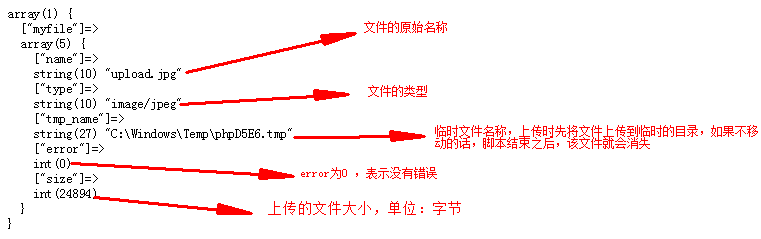# PHP文件操作实例总结【文件上传、下载、分页】

更新时间：2018年12月08日 11:20:01   转载 作者：hello_sgw

## 1、文件上传

```<!-- 例如 -->
<form action="" method="post" enctype="multipart/form-data" >
<input type="file" name="">
</form>

``````// 例如

```

### 1.2 防止上传文件过大

1GB == 1024MB
1MB == 1024KB
1KB == 1024Byte

```\$max_size = 3*1024*1024; // 约束为3M
if(\$_FILES['myfile']['size'] > \$max_size){
echo '上传图片大于3M';
exit;
}
// 上传
echo '上传成功';
} else {
echo '上传失败';
}

```

### 1.3 防止文件被覆盖

1、我们在服务器端进行验证，在移动到目的地的时候，生成新的文件名时，使用随机数命名，可以避免文件名重复
2、按日期保存文件夹

### 1.4 控制上传的文件类型

```// 为了防止用户修改文件后缀，使用php的一个扩展 Finfo 实现
# 1、开启php.ini 中php扩展
extension=php_fileinfo.dll
# 2、使用扩展类获取上传文件真实类型
\$finfo = new Finfo(FILEINFO_MIME_TYPE);
\$mime_type = \$finfo->file(\$_FILES['myfile']['tmp_name']);

```

```/*
* 说明：文件上传类
* 作者：sgw
* 时间：2018-7-31
*/
{
//成员属性
private \$_maxsize    = 2*1024*1024;  //上传的文件最大范围2M
private \$_prefix     = 'odshen_';       //文件名的前缀
private \$allow_type   = array('.jpg','.png','.gif','.jpeg');  //允许上传的文件类型
private \$allow_mime_type = array('image/jpeg','image/png','image/gif','image/jpg');
/**
* set 设置私有的属性
* @param [str] \$p [属性名]
* @param [mix] \$v [给属性赋的值]
*/
public function __set(\$p,\$v)
{
if(property_exists(\$this,\$p)){
\$this -> \$p = \$v;
}
}
/**
* get 获取私有属性
* @param [str] \$p [属性名]
*/
public function __get(\$p)
{
if(property_exists(\$this,\$p)){
return \$this -> \$p;
}
}
/**
* 上传文件方法
* @param [mix] \$file [上传的文件]
*/
{
//判断用户上传的文件大小
\$max_size = \$this->_maxsize;  //约束最大为2M
if(\$file['size'] > \$max_size){
echo '上传文件过大，重新上传';
exit;
}
#防止上传的文件被覆盖
\$prefix = \$this->_prefix;
// 文件名唯一
\$filename = uniqid(\$prefix,true);
//文件的后缀，从上传的文件名称中截取最后一个点后面的内容 strrchr
\$ext = strrchr(\$file['name'],'.');
//分目录保存上传的文件,按照日期格式保存
# 如果当前目录不存在，需要先创建 ,绝对路径
\$now_path = __DIR__.'/';
\$now_path = str_replace('\\','/',\$now_path.\$sub_path);
if(!is_dir(\$now_path)){
mkdir(\$now_path,0777,true);
}
# 限制用户上传的文件类型
if(!in_array(\$ext,\$this->allow_type)){
echo '文件类型不支持';
exit;
}
# 防止用户修改文件的后缀，借助php的一个扩展Finfo实现，精确获得每个文件在网络中的类型
\$finfo = new Finfo(FILEINFO_MIME_TYPE);
\$mime_type = \$finfo -> file(\$file['tmp_name']);
if(!in_array(\$mime_type,\$this->allow_mime_type)){
echo '文件类型不支持';
exit;
}
//参数1：待移动的文件（临时文件）
//参数2：目的地
//返回结果布尔类型
//上传成功将上传的文件地址返回，便于在其他地方使用该图片，就需要能够找到该图片
return \$sub_path . \$filename.\$ext;
}else{
//echo '上传失败';
return false;
}
}
}

```

## 2、文件下载

```//先接收地址栏中传递的文件名，也就是下载的是哪一个文件
\$filename = \$_GET['filename'];
if(\$filename==''){
echo '文件未找到，下载失败';
exit;
}
//拼接好文件的路径
\$full_name = 'img/'.\$filename;
//图片在windows系统下面gbk编码，php文件是utf-8编码
//通常需要先将php文件的编码修改为gbk
\$full_name = iconv('utf-8','gbk',\$full_name);
\$filesize = filesize(\$full_name);
//返回的文件
//按照字节大小返回
//显示文件大小
//这里客户端的弹出对话框，对应的文件名
//开始读取文件资源并回应给浏览器
\$fp = fopen(\$full_name,'r');
while(!feof(\$fp)){
echo \$data;
}
fclose(\$fp);

```

## 3、分页类

```/*
* 分页显示数据
*/
class Page
{
private \$_total;  //总的记录数
private \$_pagesize;  //每页显示的记录数
private \$_pagenow; //当前页码数
private \$_url;   //点击超链接时跳转的url地址
/**
* set 设置私有的属性
* @param [str] \$p [属性名]
* @param [mix] \$v [给属性赋的值]
*/
public function __set(\$p,\$v)
{
if(property_exists(\$this,\$p)){
\$this -> \$p = \$v;
}
}
/**
* get 获取私有属性
* @param [str] \$p [属性名]
*/
public function __get(\$p)
{
if(property_exists(\$this,\$p)){
return \$this -> \$p;
}
}
//动态创建分页导航条
public function create()
{
//定义首页按钮
//当前页高亮显示
\$first_active = \$this->_pagenow == 1?'active':'';
\$url = \$this -> _url.'?page=';
\$first = 1;
\$PAGE = <<<HTML
<ul class="pagination">
<li class="\$first_active"><a href="\$url\$first" rel="external nofollow" >首页</a></li>
HTML;
//创建中间的分页导航按钮
//先计算目前有多少页 == 总的数据 / 每页显示的数据
\$page_count = ceil(\$this -> _total / \$this ->_pagesize);
for(\$i=\$this->_pagenow-3; \$i<=\$this->_pagenow+3; \$i++){
\$active = \$this->_pagenow == \$i?'active':'';
if(\$i <= 1 || \$i>= \$page_count){
continue;  //跳出本次循环
}
\$PAGE .= <<<HTML
<li class="\$active"><a href="\$url\$i" rel="external nofollow" >\$i</a></li>
HTML;
}
//定义尾页按钮
\$last_active = \$this->_pagenow == \$page_count?'active':'';
\$PAGE .= <<<HTML
<li class="\$last_active"><a href="\$url\$page_count" rel="external nofollow" >尾页</a></li>
</ul>
HTML;
//将创建的分页导航条返回
return \$PAGE;
}
}

```